###### Descriptions and Examples for the POV-Ray Raytracerby Friedrich A. Lohmüller3D Animations with POV-Ray         Some basics and examples on animations.
Italiano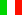Français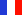Deutsch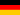Home
- 3D Animations Gallery
- POV-Ray Tutorial

3D Animation Tutorial
Index of Content
0. Basic Knowledge
1. Basic Example
2. Example 2
3. Images to Animated Gif
4. From Images to Video
5. Basic Terms
6. Animation Commands
I. Cyclic Animations
1. Rotating Objects
1.2. Planets in Orbit
1.3. Clock Animation
2. Rotating Camera
2.1. Straight Moving Camera
3. Western Wheel
Problem
3.1. Rolling Wheels
4. Gears
4.1. Roller Chain
4.2. Bike Chain
5. Swinging Pendulum
5.2: Rock the Rocker
6. Spiral Pendulum
7. Coupling Rods
7.1. Connecting Rods
8. Psychedelic + Op-Art
9. Counters + Countdowns
10. Folding of a Cube
II. Non-linear Movements
1.0 Speed Up/Slow Down 1
1.1 Speed Up/Slow Down 2
2. Fall + Bounce
> 3. Acceleration by
physical Formulas
4. Speed Controll by
Spline Functions
III. Animation Paths
with Spline Curves
1. Spline Curves
2. Closed Splines
3. Animation Paths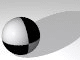# Acceleration in accordance with physical FormulasNon-linear movements in animations to simulate realistic acceleration or deceleration by physical laws of Motion with POV-Ray.

The Laws of Motion
for some simple types of motions

( t = time, s = distance, v = velocity, a = acceleration )
1. Motion with constant speed v:
 Velocity v = constant Covered distance s(t) = v * t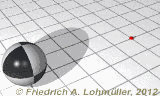2a. Motion with constant acceleration a:
 Acceleration a = constant Velocity v(t) = a * t Covered distance s(t) = 1/2*a*t2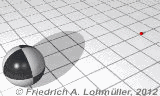2b. Motion with constant acceleration
+ initial velocity v0:

 Acceleration a = constant Velocity v(t) = a * t + v0 Covered distance s(t) = 1/2*a*t2 + v0*t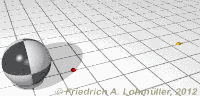Simulation of an motion with acceleration a
and with constant initial velocity v0:
 ```//--------------------------------------------------- #declare Total_Time = 4.48; #declare Time = Total_Time*clock; // #declare Time_A = 2.65; // start acceleration #declare Time_B = 3.45; // end acceleration // total acceleration time: #declare Ac_Time = Time_B-Time_A; // last sector without acceleration: #declare End_Time= Total_Time-Time_B; #declare Acceleration = 5.0 ; // v(0) = constant speed at start: #declare V_0 = 1 ; // final speed after acceleration #declare End_Speed = Acceleration * Ac_Time + V_0 ; // covered distances //1: with constant speed: #declare Way1 = V_0*Time_A ; //2: with constant acceleration #declare Way2 = Acceleration/2*pow(Ac_Time,2)+V_0*Ac_Time+Way1; //note: pow(Ac_Time,2) = Ac_Time*Ac_Time // with constant speed in last sector //3: total distance // --- distance totale #declare Way3 = End_Speed*( End_Time ) + Way2 ; //---------------------------------------------------```

###### Scene descriptions for POV-Ray: "Accelerate_speed_1.ini" and "Accelerate_speed_1.pov"
Calculation for the covered distance
 ```//--------------------------------------------------- // calculating the distance at 'Time' // --- calulation de la distance au temps 'Time' // covered by the rolling sphere; // --- parcourue de la sphère roulante: // constant translation #if ( Time < Time_A ) #declare Way = V_0*Time; #end // constant translation + acceleration #if (( Time >= Time_A ) & ( Time < Time_B )) #declare Way = Acceleration/2*pow(Time - Time_A,2) + V_0*(Time - Time_A) + Way1; #end // way with constant speed after acceleration ends #if ( Time >= Time_B ) #declare Way = End_Speed*( Time - Time_B) + Way2; #end //--------------------------------------------------- sphere{<0,0,0>,0.75 translate<0,0.75, Way>} //---------------------------------------------------```
About the rolling of the sphere see the scene descriptions!
top